Next: Miranda theorem Up: Introduction Previous: Implementation   Contents

Interval Newton

The classical interval Newton method is embedded in the procedure GradientSolve and HessianSolve but may also be useful in other procedures. Furthermore this method relies on the use of the product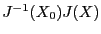where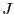is the Jacobian of the system of equations and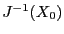the inverse ofcomputed at some particular point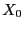. In the classical method this product is cimputed numerically and this does not take into account that the element ofare functions of the same parameters. For example if the first column ofis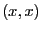where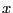is some parameter with interval value, the first element ofwill be computer as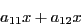where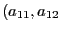are the elements of. Clearly the double occurence ofin the numerical evaluation of the elements may lead to an overestimation of the elements: this element should be written as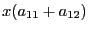which is optimal in term of interval evaluation. Furthermore it may also be interesting to have the derivatives of each element of the product in order to improve the interval evalation of the matrix product. Indeed the interval evaluation ofplays a very important role in the interval Newton method either for filtering a box for possible solution or for determining that a box includes a solution of the system.

The procedure IntervalNewton is a sophisticated interval Newton algorithm that allows one to introduce knowledge on the product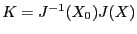in the classical scheme. Its syntax is:

int IntervalNewton(int Dim,INTERVAL_VECTOR &P,INTERVAL_VECTOR &FDIM,
where
• Dim: the size of the system
• P: an interval vector that describes the range for the unknowns
• FDIM: interval value of the equation at the mid-point of P
• Grad: interval jacobian at P
• GradMid: jacobian at the mid-point of P
• hasBgrad: a flag that indicates how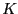will be calculated:
• 0:will be calculated numerically
• 1:will be calculated using the procedure BgradFunc
• 2:will be calculated using the procedure BgradFunc and the derivatives of the elements ofavailable through the procedure BgradJFunc
• BgradFunc: a user-provided procedure in MakeF format that calculate the element of, row by row
• BgradJFunc: a user-provided procedure in MakeJ format that calculate the derivatives of the elements of• grad1: if hasBgrad is 1 or 2 we use the procedure BgradFunc to evaluatewhen we are in the 3B filter if grad1 is set to 1. If set to 0 we use BgradFunc only when dealing with the full box
• grad3B1: if 1 we use the procedure that evaluatesthrough BgradFunc even if we are in the 3B case. If 2 we use both BgradFunc and BgradJFunc
The procedure returns -1 if no solution of the system exists in P, 1 if P has been improved, 0 otherwise. Note that the procedure BgradFunc and BgradJFunc may require the availability of the mid-matrix GradMid: therefore a global MATRIX should be made available, initialized with GradMid.

Various variants of IntervalNewton are available:

int IntervalNewton(int Dim,INTERVAL_VECTOR &P,INTERVAL_VECTOR &FMID,

int IntervalNewton(int Dim,INTERVAL_VECTOR &P,int DimVar,int DimEq,
INTERVAL_VECTOR (*TheIntervalFunction)(int,int,INTERVAL_VECTOR &),
INTERVAL_MATRIX (* Gradient)(int, int, INTERVAL_VECTOR &))
is also the classical interval Newton method for a system having DimVar unknowns and DimEq equations (here DimVar and DimEq are not required to have the same value: only the Dim first equations will be considered). The flag TypeGradMid is used to determine how the mid jacobian matrix is calculated: if 0 this matrix is calculated for the mid-point of P, if 1 the mid-jacobian is calculated as the mid-matrix of the interval jacobian calculated for P.

int IntervalNewton(int Dim,INTERVAL_VECTOR &P,int DimEq,int DimVar,